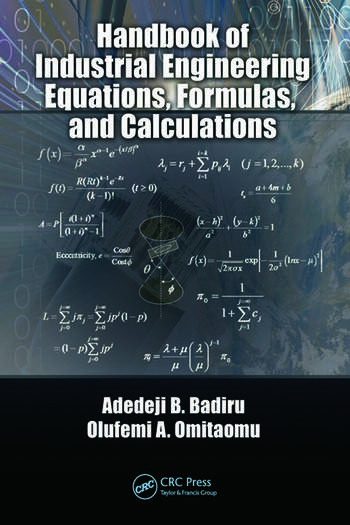## Monday, December 3, 2018

### Handbook of Industrial Engineering Equations, Formulas, and Calculations (Free PDF)File Size: 3.09 mb

Description
The first handbook to focus exclusively on industrial engineering calculations with a correlation to applications, Handbook of Industrial Engineering Equations, Formulas, and Calculations contains a general collection of the mathematical equations often used in the practice of industrial engineering. Many books cover individual areas of engineering and some cover all areas, but none covers industrial engineering specifically, nor do they highlight topics such as project management, materials, and systems engineering from an integrated viewpoint. Written by acclaimed researchers and authors, this concise reference marries theory and practice, making it a versatile and flexible resource. Succinctly formatted for functionality, the book presents: Basic Math Calculations; Engineering Math Calculations; Production Engineering Calculations; Engineering Economics Calculations; Ergonomics Calculations; Facility Layout Calculations; Production Sequencing and Scheduling Calculations; Systems Engineering Calculations; Data Engineering Calculations; Project Engineering Calculations; and Simulation and Statistical Equations. It has been said that engineers make things while industrial engineers make things better. To make something better requires an understanding of its basic characteristics and the underlying equations and calculations that facilitate that understanding. To do this, however, you do not have to be computational experts; you just have to know where to get the computational resources that are needed. This book elucidates the underlying equations that facilitate the understanding required to improve design processes, continuously improving the answer to the age-old question: What is the best way to do a job?

Content:-
1. Computational Foundations of Industrial Engineering
2. Basic Mathematical Calculations
3. Statistical Distributions, Methods, and Applications
4. Computations with Descriptive Statistics
5. Computations for Economic Analysis
6. Industrial Production Calculations
7. Forecasting Calculations
8. Six Sigma and Lean
9. Risk Computations
10. Computations for Project Analysis
11. Product Shape and Geometrical Calculations
12. General Engineering Calculations
Appendix A: Mathematical Patterns, Series, and Formulae
Appendix B: Measurement Units, Notation, and Constants
Appendix C: Conversion Factors
Appendix D: Factors and Tables
Bibliography
Index

Author Details
"Adedeji Badiru" is a professor and head of the Department of Systems and Engineering Management at the Air Force Institute of Technology, Dayton, Ohio. He was previously professor and head of the Department of Industrial and Information Engineering at the University of Tennessee in Knoxville, Tennessee. Prior to that, he was professor of Industrial Engineering and Dean of University College at the University of Oklahoma. He is a registered professional engineer (PE), a certified project management professional (PMP), a fellow of the Institute of Industrial Engineers, and a fellow of the Nigerian Academy of Engineering.

"Olufemi Omitaomu" is a research scientist in the Computational Sciences and Engineering Division at the Oak Ridge National Laboratory, Tennessee, and Adjunct assistant professor in the Department of Industrial and Information Engineering at the University of Tennessee, Knoxville, Tennessee. He holds BS in Mechanical Engineering from Lagos State University, Nigeria, MS in Mechanical Engineering from the University of Lagos, Nigeria, and PhD in Industrial and Information Engineering from the University of Tennessee.